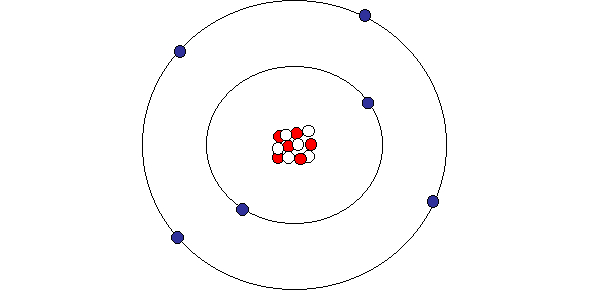# Atomic Structure Quiz: MCQ Trivia!

31 Questions | Attempts: 349Settings• 1.
What is the chemical symbol for calcium?
• A.

Cl

• B.

C

• C.

Ca

• D.

Cu

• E.

Cr

• 2.
What is the atomic mass of phosphorus?
• A.

15

• B.

31

• C.

2

• D.

18

• E.

16

• 3.
What type of element is Potassium?
• A.

Metal

• B.

Non-metal

• C.

Metalloid

• D.

Noble Gas

• E.

Halogen

• 4.
A positively charged subatomic particle is?
• A.

Proton

• B.

Mass Number

• C.

Element Number

• D.

Neutron

• 5.
The atom oxygen has a neucleon number of 16 and a proton number of 8. what is the number of neutrons in the oxygen atom?
• A.

4

• B.

16

• C.

8

• D.

0

• 6.
An atom has very little empty space.
• A.

True

• B.

False

• 7.
The number of protons in an atom is known as the?
• A.

Proton number

• B.

Atomic number

• C.

Mass number

• D.

Atomic mass

• 8.
This subatomic particle has a negative charge.
• A.

Neutron

• B.

Electron

• C.

Proton

• D.

Isotope

• 9.
This subatomic particle has a positive charge.
• A.

Proton

• B.

Electron

• C.

Neutron

• D.

Atom

• 10.
How many neutrons does an atom with an atomic number of 23 and a mass number of 51 have?
• A.

23

• B.

28

• C.

51

• D.

74

• 11.
Electrons are found:
• A.

Inside nucleus

• B.

Outside nucleus

• C.

With protons

• D.

With neutrons

• 12.
The ? and ? are found in the nuclues.
• A.

Electrons and neutron

• B.

Protons and electrons

• C.

Protons and neutrons

• D.

Protons and electrons

• 13.
These particles make up the mass of an atom.
• A.

Protons and neutrons

• B.

Electrons and neutrons

• C.

Protons and electrons

• 14.
The element chlorine has an electron configuration of 2,8,7. It is in which group and period?
• A.

Group 7 and period 3

• B.

Group 17 and period 3

• C.

Group 3 and period 17

• D.

Group 3 and period 7

• 15.
Atoms are arranged in the periodic table according to increasing numbers of?
• A.

Neutrons

• B.

Electrons

• C.

Protons

• D.

Nuclei

• 16.
The symbol for sodium is?
• A.

So

• B.

S

• C.

N

• D.

Na

• 17.
An atom has very little empty space.
• A.

True

• B.

False

• 18.
The number of protons in an atom is known as the?
• A.

Proton number

• B.

Atomic number

• C.

Mass number

• D.

Atomic mass

• 19.
This subatomic particle has a negative charge.
• A.

Neutron

• B.

Electron

• C.

Proton

• D.

Isotope

• 20.
This subatomic particle has a positive charge.
• A.

Proton

• B.

Electron

• C.

Neutron

• D.

Atom

• 21.
How many neutrons does an atom with an atomic number of 23 and a mass number of 51 have?
• A.

23

• B.

28

• C.

51

• D.

74

• 22.
Electrons are found?
• A.

Inside nucleus

• B.

Outside nucleus

• C.

With protons

• D.

With neutrons

• 23.
The ? and ? are found in the nucleus.
• A.

Electrons and neutron

• B.

Protons and electrons

• C.

Protons and neutrons

• D.

Protons and electrons

• 24.
These particles make up the mass of an atom.
• A.

Protons and neutrons

• B.

Electrons and neutrons

• C.

Protons and electrons

• 25.
The element chlorine has an electron configuration of 2,8,7. It is in which group and period?
• A.

Group 7 and period 3

• B.

Group 17 and period 3

• C.

Group 3 and period 17

• D.

Group 3 and period 7

## Related TopicsBack to top
×

Wait!
Here's an interesting quiz for you.